Economic Order Quantity (EOQ): Definition

The economic order quantity (EOQ) is the specific total order amount for a firm’s inventory that minimizes the total cost of inventory management.

That is to say, EOQ refers to the size of the order that gives the maximum economy when purchasing any material. It is the optimal standard ordering quantity.

The EOQ is also commonly referred to as the economic lot quantity or economic buying quantity.

EOQ: Explanation

The concept of EOQ relates to the quantity of materials that a purchasing department aims to procure.

The EOQ is the size of the order that yields the best economy when purchasing any material. To determine the optimal order quantity, an analysis is undertaken of the various costs associated with the order quantity. These costs are divided into two parts:

• Material acquisition costs
• Material carrying costs

Material acquisition costs arise on account of having to process an order. Part of the wages and operating expenses for departments such as production control, purchasing, receiving, and storage are incurred for purchasing and possessing the materials.

Carrying costs for materials, or material carrying costs, include interest charges on the investment in materials, insurance costs, storage costs, and other factors.

It is worth noting that material acquisition costs and material carrying costs behave quite differently.

Material acquisition costs are related to the number of orders placed during a given period. On the other hand, carrying costs, which are variable or semi-variable in nature, tend to change nearly in direct proportion to the level of stock carried in a manufacturing firm.

Purchasing or buying in large quantities implies low acquisition or purchasing costs (i.e., the number of purchase orders placed with the suppliers is small) and high carrying costs. This is due to the amount of stock carried during this period being greater.

On the other hand, purchasing in small quantities implies high purchasing or acquisition costs and low carrying costs.

The most advantageous, or economic, quantity (i.e., the EOQ) will be at a point where the acquisition costs and the costs of carrying stock are equal; namely, where total costs to order and to carry a given material are minimum.

Two important categories of inventory costs are ordering costs and carrying costs. Ordering costs are costs that are incurred to obtain additional inventories, whereas carrying costs are the costs incurred to hold inventory on hand.

The total inventory cost is the ordering cost plus the carrying cost. It is necessary to minimize total inventory costs, and the EOQ concept is ideal in helping to achieve this. Specifically, the EOQ formula shown in the next section should be applied.

EOQ Formula

The common formula used to compute the economic order quantity (EOQ) is: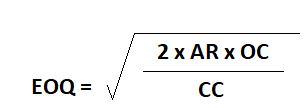where:

• AR =  Annual requirements
• OC = Per unit cost
• CC = Carrying cost per unit per year of materials inventory

Factors Governing the Fixation of EOQ

The following factors govern the fixation of EOQ:

• Procurement cost
• Carrying cost

An overview of these costs is given in the next sections.

Procurement cost

The cost of obtaining quotations, preparing/placing orders, conducting follow-up actions, receiving and inspecting, and paying bills are all included in the procurement cost. This is also known as the cost of acquisition.

The salaries of the personnel involved in all business processes are the main items to be considered when calculating the procurement cost.

For a single order, the procurement cost is the total cost, which is obtained by adding up all the above costs and dividing the result by the number of orders placed during that period.

To determine the procurement cost of multi-item orders, the total cost is divided by the number of items ordered during a given period of time.

Carrying cost

Carrying cost is calculated based on the insurance premium, depreciation, obsolescence, storage, preservation costs, and interest on value of stock held, including that of handling and other allied costs. This cost is also known as the possession cost.

These costs vary from item to item and plant to plant. Average carrying cost is taken as 15% to 25% of the value of average working cost, which usually amounts to 50% of the order quantity.

Example

The XYZ Equipment Company estimates its carrying cost at 15% and its ordering cost at \$9 per order. The estimated annual requirement is 48,000 units at a price of \$4 per unit.

1. What is the most economical no. of units to order?
2. How many orders should be placed in a year?
3. How often should orders be placed?
4. What is the ordering cost?
5. What is the carrying cost?
6. What is the combined ordering and holding cost at the EOQ?

Solution

1. No. of units to order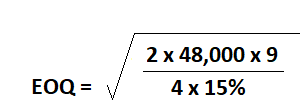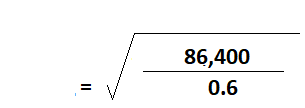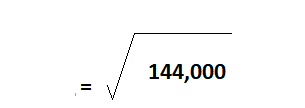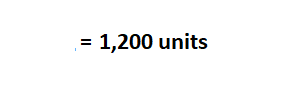2. No. of orders to be placed in a year
No. of orders per year = Annual requirement / EOQ
= 48,000 / 1,200
= 40 orders
3. How often will an order need to be placed? (i.e., frequency of orders)
Frequency of orders = No. of days in one year / No. of orders
= 360 / 40
= 9 days
4. Ordering cost
= No. of orders per year x Cost per order
= 40 x 9
= \$360
5. Carrying cost
= Average units x Carrying cost per unit
= (1,200/ 2) x 1.35
= 600 x 1.35
= \$810
6. Combined ordering and holding cost at the EOQ
= Ordering cost + Carrying cost
= \$360 + \$810
= \$1,170

True is a Certified Educator in Personal Finance (CEPF®), contributes to his financial education site, Finance Strategists, and has spoken to various financial communities such as the CFA Institute, as well as university students like his Alma mater, Biola University, where he received a bachelor of science in business and data analytics.

To learn more about True, visit his personal website, view his author profile on Amazon, his interview on CBS, or check out his speaker profile on the CFA Institute website.